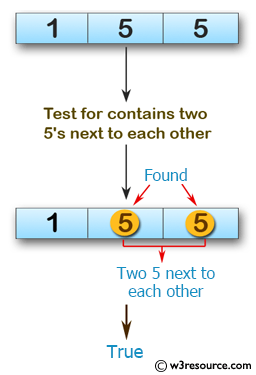﻿ Swift Array Programming Exercise: Test if a given array of integers contains two 5's next to each other - w3resource# Swift Array Programming Exercises: Test if a given array of integers contains two 5's next to each other

## Swift Array Programming: Exercise-32 with Solution

Write a Swift program to test if a given array of integers contains two 5's next to each other, or there are two 5's separated by one element.

Example: {5, 5, 1}, {5, 1, 5}

Pictorial Presentation:Sample Solution:

Swift Code:

``````func num55(array_nums: [Int]) -> Bool {
for x in 0..<array_nums.count-1
{
if (array_nums[x] == 5 && array_nums[x+1] == 5) || (array_nums[x] == 5 && array_nums[x+2] == 5)
{
return true
}
}
return false
}
print(num55(array_nums: [1, 5, 5]))
print(num55(array_nums: [1, 5, 1, 5]))
print(num55(array_nums: [1, 5, 1, 1, 5]))
```
```

Sample Output:

```true
true
false
```

Swift Programming Code Editor:

Improve this sample solution and post your code through Disqus

What is the difficulty level of this exercise?

﻿